How Cheenta works to ensure student success?
Explore the Back-Story

# Test of Mathematics Solution Subjective 175 - Integer RootsThis is a Test of Mathematics Solution Subjective 175 (from ISI Entrance). The book, Test of Mathematics at 10+2 Level is Published by East West Press. This problem book is indispensable for the preparation of I.S.I. B.Stat and B.Math Entrance.

Also visit: I.S.I. & C.M.I. Entrance Course of Cheenta

## Problem

Let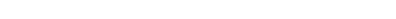be a polynomial with integer coefficients,such that,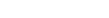are odd integers.Show that

(a)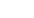does not have any even integer roots.

(b)does not have any odd integer roots.

## Solution

Given the two statements (a) and (b)  above it is clear that if we can prove thathas no integer roots,then we are done.

Let us assumehas an integer root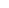.

Then we can write,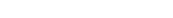where,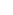is any function of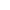.

Now ,putting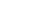in,we get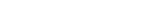and,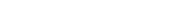now as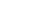and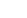,are consecutive integers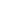andcannot be both odd,

which means that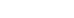cannot be both odd,given whatever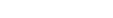are.

So,we can conclude that there exists no integer solution of.

Hence,we are done.This is a Test of Mathematics Solution Subjective 175 (from ISI Entrance). The book, Test of Mathematics at 10+2 Level is Published by East West Press. This problem book is indispensable for the preparation of I.S.I. B.Stat and B.Math Entrance.

Also visit: I.S.I. & C.M.I. Entrance Course of Cheenta

## Problem

Letbe a polynomial with integer coefficients,such that,are odd integers.Show that

(a)does not have any even integer roots.

(b)does not have any odd integer roots.

## Solution

Given the two statements (a) and (b)  above it is clear that if we can prove thathas no integer roots,then we are done.

Let us assumehas an integer root.

Then we can write,where,is any function of.

Now ,puttingin,we getand,now asand,are consecutive integersandcannot be both odd,

which means thatcannot be both odd,given whateverare.

So,we can conclude that there exists no integer solution of.

Hence,we are done.

### Knowledge Partner